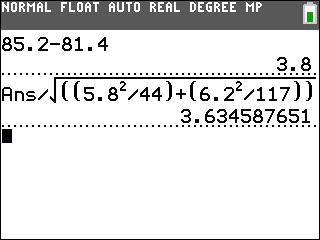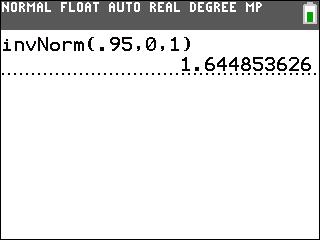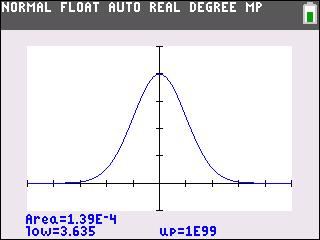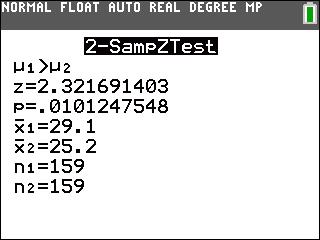# Activities

••• ##### Subject Area

• Math: Statistics: Hypothesis Tests

• ##### Author9-12

40 Minutes

• ##### Device
• TI-83 Plus Family
• TI-84 Plus
• TI-84 Plus Silver Edition
•TI-84 Plus C Silver Edition
•TI-84 Plus CE
• ##### Report an Issue

Comparing Two Means#### Activity Overview

Students test hypotheses concerning means of two populations by calculating the test statistic and the critical values and graphing the critical region.

#### Key Steps

•Students calculate the test statistic using the given formula on the worksheet. They will determine the critical value by using the invNorm command from the DISTR menu.

•Comparing the test statistic and critical value, students decide whether to reject or fail to reject the null hypothesis. Then they use the ShadeNorm command to find the P-value, confirming their decision about the hypothesis.

•At the end of this activity, students will be able to compare two means for a real-life scenario. They calculate the test statistics, critical values, and P-values. They will also learn to use the 2-SampZTest command to calculate the P-value instead of the ShadeNorm command.